概述

常见的八大排序算法，他们之间关系如下：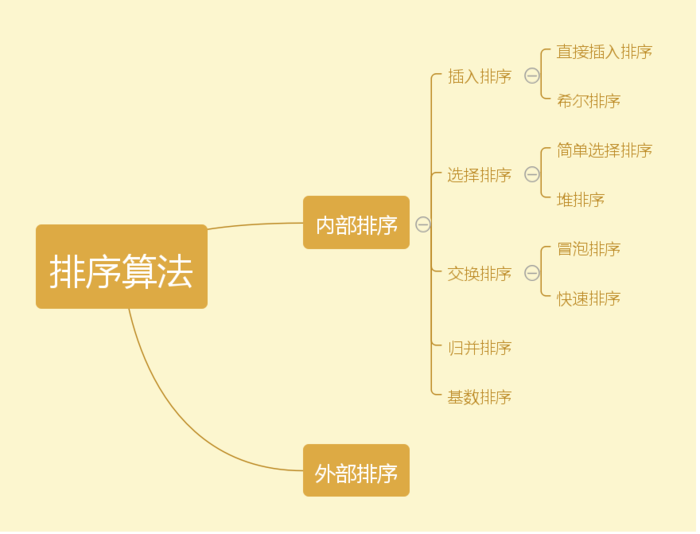本文的开发语言是用Java，为了更好的演示，这里先新建一个工具类NumberUtils ，用来生成随机数组和打印排序前后数组的内容。

``````public class NumberUtils {
/** * 获取随机int类型数组 */
public static int[] getRandomArs(int length,int max){
int rs[]=new int[length];
Random random=new Random();
for(int i=0;i<length;i++){
rs[i]=random.nextInt(max);
}
return rs ;
}
/** * 打印内容 */
public static void display(int intArrays[],int type){
int count=0;
if(type==2){
System.out.print("排序后：");
}else if(type==1){
System.out.print("排序前：");
}
if(intArrays.length<40){
for(int i:intArrays){
System.out.print(i+" ");
}
}else {
for(int i:intArrays){
count++;
if(count<10){
System.out.print(i+" ");
}else if(count==10){
System.out.print("......");
}else if(count> intArrays.length-10){
System.out.print(i+" ");
}
}
}
System.out.println();
}

/** * 交换数组中两个数的位置 */
public static void exchange(int[] a, int i, int j) {
int temp = a[i];
a[i] = a[j];
a[j] = temp;
}
}``````

插入排序

直接插入排序

直接插入排序是一种简单插入排序，基本思想是：把n个待排序的元素看成为一个有序表和一个无序表。开始时有序表中只包含1个元素，无序表中包含有n-1个元素，排序过程中每次从无序表中取出第一个元素，将它插入到有序表中的适当位置，使之成为新的有序表，重复n-1次可完成排序过程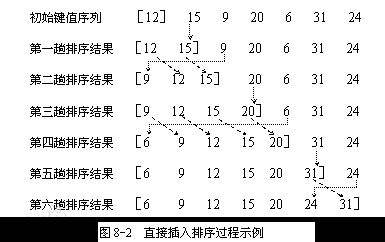类似我们摸牌，一开始有一堆牌（待排序的）。由于第一次摸牌时手中没牌，所以不需要排序。第二次摸牌时和手中第一张拍比较，如果它大，就放在它的后面。 每次摸牌都会把牌放在一个前面比自己小（或等于），后面比自己大（或等于）的位置。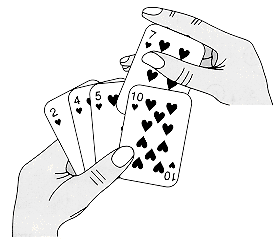直接插入排序的Java代码

`````` public class Insertion {

/** * 直接插入排序算法方法 */
public static void sort(int intArrays[]){
int length=intArrays.length;
int i,j;
for(i=1;i<length;i++){
for(j=i-1;j>=0&&intArrays[i]<intArrays[j];j--){
//intArrays[i]代表要插入的数字，intArrays[j]代表需要比较大小的数字，j递减
//当intArrays[i]大于intArrays[j]时（intArrays[i]插入的位置，也就是说插在j的后一位）或者intArrays[i]为当前数组的最小值时（此时的j为-1,也就是说intArrays[i]要插在第一位）返回j
}
//将intArrays[i]保存住，因为要j以后的数组向后移一位
int temp=intArrays[i];
for(int k=i;k>j+1;k--){
//将i到j范围的数组向后移一位
intArrays[k]=intArrays[k-1];
}
//intArrays[i]插在j的后一位
intArrays[j+1]=temp;
}
}

/** * 执行入口，intArrays：待排序的数组，displaySort：是否显示排序前和排序后的内容 */
public static void run(int intArrays[],boolean displaySort){
int arrays[]= intArrays.clone();
if(displaySort){
NumberUtils.display(arrays,1);
}
long startTime=System.currentTimeMillis();
sort(arrays);
long endTime=System.currentTimeMillis();
if(displaySort){
NumberUtils.display(arrays,2);
}
System.out.println("插入排序用时："+(endTime-startTime)+"毫秒");
}
/** * 测试排序用的主方法 */
public static void main(String[] args){
//数组长度
int length=30000;
//最大值
int max =100000000;
//是否打印排序后的内容
boolean display=true;
//随机获取的排序数组
int intArrays[]= NumberUtils.getRandomArs(length,max);
//插入排序
Insertion.run(intArrays,display);
}
}``````

运行main方法，终端输出

``````排序前：52096862 89211005 17092175 60370534 40302430 53897879 34127111 43318378 29195095 ......44543512 40238517 99995921 49109471 44841738 2383202 81685348 82456235 16748589 90004692

希尔排序

该方法的基本思想是：先将整个待排元素序列分割成若干个子序列（由相隔某个“增量”的元素组成的）分别进行直接插入排序，然后依次缩减增量再进行排序，待整个序列中的元素基本有序（增量足够小）时，再对全体元素进行一次直接插入排序。因为直接插入排序在元素基本有序的情况下（接近最好情况），效率是很高的，因此希尔排序在时间效率上比前两种方法有较大提高。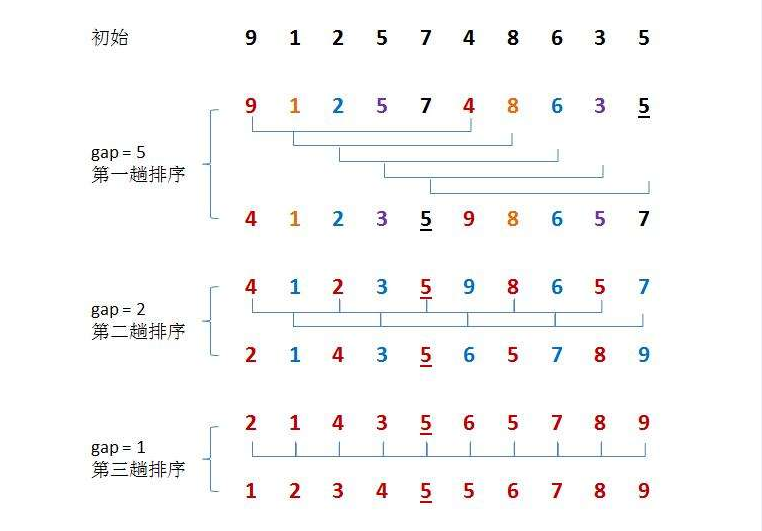希尔排序的Java代码

``````public class Shell {
public static void sort(int[] intArrays) {
int length = intArrays.length;
int h = 1;
int block=3;//分块大小（大于1的值）
//h为分区后每块有多少个元素
while (h < length / block) {
h = block* h + 1; //通过循环算出h的取值，当分区大小为3时，h序列为1, 4, 13, 40, ...
}
while (h >= 1) {
int n, i ,j, k;
//分割后，产生n个子序列
for (n = 0; n < h; n++) {
//分别对每个子序列进行直接插入排序
for (i = n + h; i < length; i += h) {
for (j = i - h; j >= 0 && intArrays[i] < intArrays[j]; j -= h) {

}
int tmp = intArrays[i];
for (k = i; k > j + h; k -= h) {
intArrays[k] = intArrays[k-h];
}
intArrays[j+h] = tmp;
}
}
//直接插入排序完后，减少每块区里的元素。也就是说增大块区的数量，直到最后h=1(每块区里只有一个元素时，排序完成)
h = h / block;
}
}
/** * 执行入口，intArrays：待排序的数组，displaySort：是否显示排序前和排序后的内容。 */
public static void run(int intArrays[],boolean displaySort){
//克隆一份数组
int arrays[]= intArrays.clone();
// 判断是否需要显示排序前的内容
if(displaySort){
NumberUtils.display(arrays,1);
}
// 记录开始时间
long startTime=System.currentTimeMillis();
sort(arrays);
// 记录结束时间
long endTime=System.currentTimeMillis();
// 判断是否需要显示排序前的内容
if(displaySort){
NumberUtils.display(arrays,2);
}
System.out.println("希尔排序用时："+(endTime-startTime)+"毫秒");
}
/** * 测试排序用的主方法 */
public static void main(String[] args){
//数组长度
int length=30000;
//最大值
int max =100000000;
//是否打印排序后的内容
boolean display=true;
//随机获取的排序数组
int intArrays[]= NumberUtils.getRandomArs(length,max);
//希尔排序
Shell.run(intArrays,display);

}
}``````

运行main方法，终端输出

``````排序前：5899233 16208367 81634733 42495727 1153823 76149452 14140770 48392747 88266082 ......25538919 94969077 11926461 62607362 11544024 8288043 43560779 20729207 53683969 31870096

选择排序

简单选择排序

从待排序序列中，找到关键字最小的元素，如果最小元素不是待排序序列的第一个元素，将其和第一个元素互换。从余下的 N - 1 个元素中，找出关键字最小的元素，重复(1)、(2)步，直到排序结束。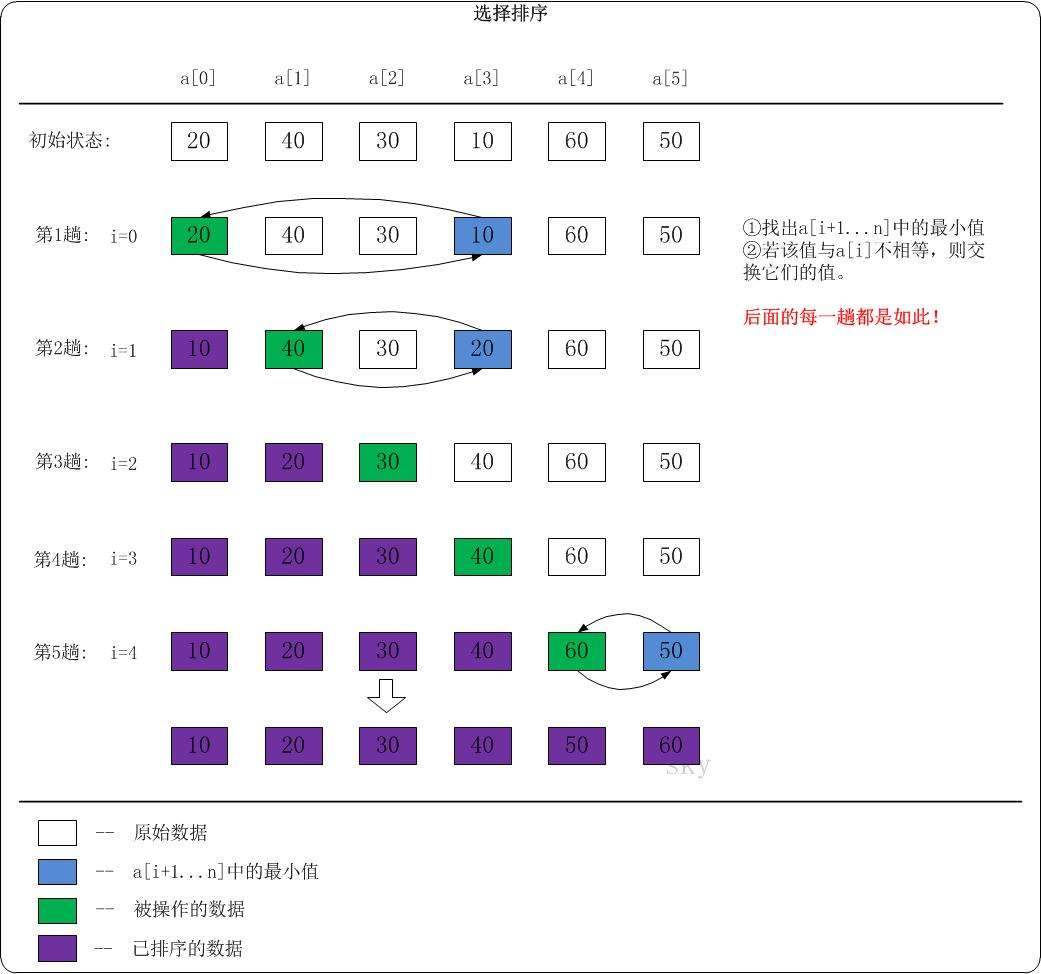选择排序的Java代码：

``````public class Selection {
public static void sort(int intArrays[]){
int length=intArrays.length;//数组长度
//循环比较，假如数组长度为10，则循环9次。第一趟循环有10个数，找出这十个数的最小值放在一位。第二次循环找出另外9个数的最小值，放在数字第二位。第三次找出另外8个数最小值，以此类推...
for(int i=0;i<length-1;i++){
//用来保存每一趟最小值数组的下标，开始前假设第一个数字为这趟的最小值
int minIndex=i;
//找出每一趟的最小值，如果有最小值就将这趟的最小值放在这趟数组第一个位，如果没有最小值就继续执行下一趟
for(int j=i+1;j<length;j++){
if(intArrays[j]<intArrays[minIndex]){
//如果这一趟有最小值,则保存它的下标。如果这一趟没有最小值，这下标还是这趟的第一个数字
minIndex=j;
}
}
//将这趟的第一个数字和这趟的最小值交换位置
NumberUtils.exchange(intArrays,i,minIndex);
}

}

/** * 执行入口，intArrays：待排序的数组，displaySort：是否显示排序前和排序后的内容。 */
public static void run(int intArrays[],boolean displaySort){
//克隆一份数组
int arrays[]= intArrays.clone();
// 判断是否需要显示排序前的内容
if(displaySort){
NumberUtils.display(arrays,1);
}
// 记录开始时间
long startTime=System.currentTimeMillis();
sort(arrays);
// 记录结束时间
long endTime=System.currentTimeMillis();
// 判断是否需要显示排序前的内容
if(displaySort){
NumberUtils.display(arrays,2);
}
System.out.println("选择排序用时："+(endTime-startTime)+"毫秒");
}
/** * 测试排序用的主方法 */
public static void main(String[] args){
//数组长度
int length=30000;
//最大值
int max =100000000;
//是否打印排序后的内容
boolean display=true;
//随机获取的排序数组
int intArrays[]= NumberUtils.getRandomArs(length,max);
//选择排序
Selection.run(intArrays,display);
}
}``````

运行main方法，终端输出

``````排序前：36256124 88245266 79330698 29894836 61606201 15293028 79866040 83062619 56056393 ......91896916 65206360 93030472 15057437 67731366 10136030 26583447 91575080 26075174 23165997

堆排序

什么是堆？
堆是一棵顺序存储的完全二叉树。

(1) Ri <= R2i+1 且 Ri <= R2i+2 (小根堆)
(2) Ri >= R2i+1 且 Ri >= R2i+2 (大根堆)
利用大顶堆(小顶堆)堆顶记录的是最大关键字(最小关键字)这一特性，使得每次从无序中选择最大记录(最小记录)变得简单。
其基本思想为(大顶堆)：
1)将初始待排序关键字序列(R1,R2….Rn)构建成大顶堆，此堆为初始的无序区；
2)将堆顶元素R与最后一个元素R[n]交换，此时得到新的无序区(R1,R2,……Rn-1)和新的有序区(Rn),且满足R[1,2…n-1]<=R[n];
3)由于交换后新的堆顶R可能违反堆的性质，因此需要对当前无序区(R1,R2,……Rn-1)调整为新堆，然后再次将R与无序区最后一个元素交换，得到新的无序区(R1,R2….Rn-2)和新的有序区(Rn-1,Rn)。不断重复此过程直到有序区的元素个数为n-1，则整个排序过程完成。
构建初始堆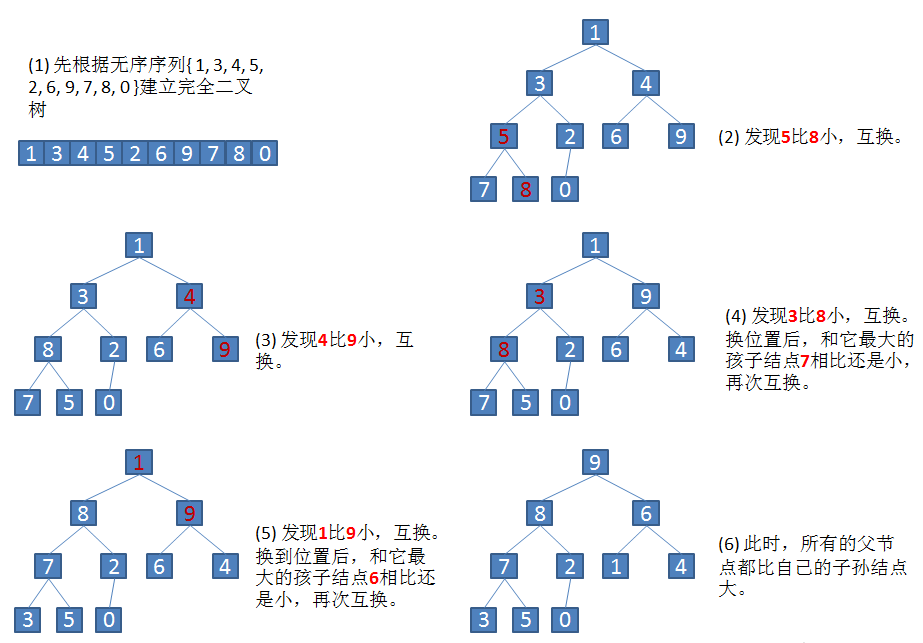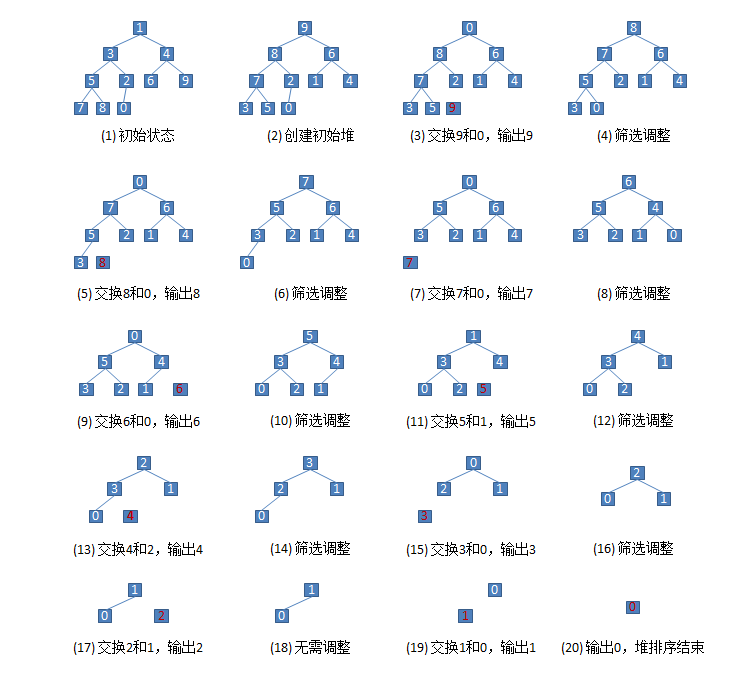堆排序的java代码

``````    public class Heap {
/** * 交换第一个和最后一个元素，输出最后一个元素（最大值），然后把剩下元素重新调整为大根堆 */
public static void sort(int[] array, int parent, int length) {
int temp = array[parent]; // temp保存当前父节点
int child = 2 * parent + 1; // 先获得左孩子
while (child < length) {
// 如果有右孩子结点，并且右孩子结点的值大于左孩子结点，则选取右孩子结点
if (child + 1 < length && array[child] < array[child + 1]) {
child++;
}
// 如果父结点的值已经大于孩子结点的值，则直接结束

if (temp >= array[child]){
break;
}
// 把孩子结点的值赋给父结点
array[parent] = array[child];
// 选取孩子结点的左孩子结点,继续向下筛选
parent = child;
child = 2 * child + 1;
}
array[parent] = temp;
}
/** * 堆排序开始入口 */
public static void sort(int[] list) {
// 循环建立初始堆
for (int i = list.length / 2; i >= 0; i--) {
sort(list, i, list.length);
}
// 进行n-1次循环，完成排序
for (int i = list.length - 1; i > 0; i--) {
// 最后一个元素和第一元素进行交换
int temp = list[i];
list[i] = list;
list = temp;
// 筛选 R 结点，得到i-1个结点的堆
sort(list, 0, i);
}
}
/** * 执行入口，intArrays：待排序的数组，displaySort：是否显示排序前和排序后的内容。 * @Author linyuanhuang */
public static void run(int intArrays[],boolean displaySort){
//克隆一份数组
int arrays[]= intArrays.clone();
// 判断是否需要显示排序前的内容
if(displaySort){
NumberUtils.display(arrays,1);
}
// 记录开始时间
long startTime=System.currentTimeMillis();
sort(arrays);
// 记录结束时间
long endTime=System.currentTimeMillis();
// 判断是否需要显示排序前的内容
if(displaySort){
NumberUtils.display(arrays,2);
}
System.out.println("堆排序用时："+(endTime-startTime)+"毫秒");
}
/** * 测试排序用的主方法 */
public static void main(String[] args){
//数组长度
int length=30000;
//最大值
int max =100000000;
//是否打印排序后的内容
boolean display=true;
//随机获取的排序数组
int intArrays[]= NumberUtils.getRandomArs(length,max);
//堆排序
Heap.run(intArrays,display);
}
}``````

运行main()方法，终端输出

``````排序前：68501387 84285957 90673930 28384601 15869847 64540413 10108132 30787337 38015080 ......12618940 19612007 61253848 71654829 94083159 68570180 32741260 91442376 31312672 25760202

交换排序

冒泡排序

它适合数据规模很小的时候，而且它的效率也比较低，但是作为入门的排序算法，还是值得学习的。
什么是冒泡排序？
顾名思义，像水里吐的泡泡一样，因为水越深压强越大，而泡泡的在水里的由深变浅。所以，同样的气体体积，第一个出来的泡泡比第二个出来的要大。如下图所示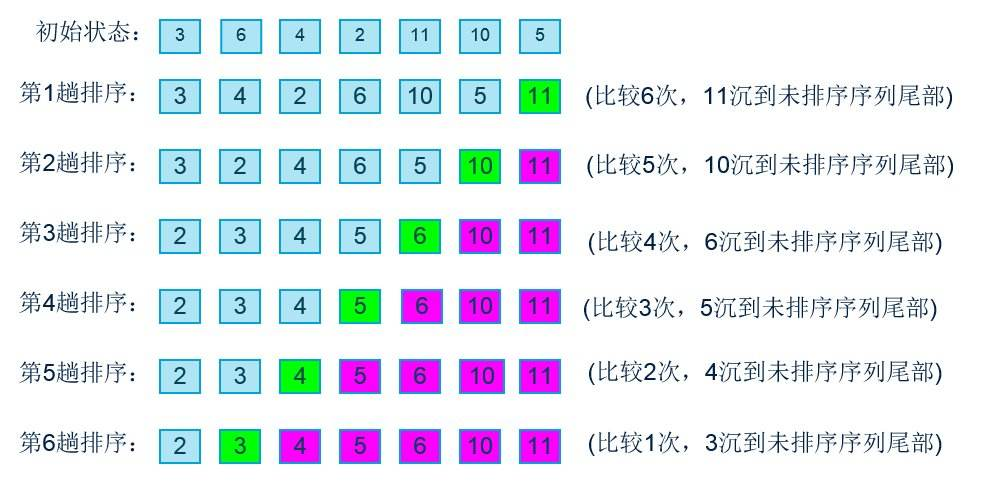冒泡排序的Java代码

``````public class Bubble {

/** * 冒泡排序算法方法，intArrays为传入的数组 */
public static void sort(int[] intArrays){
int length=intArrays.length-1;
for(int i=0;i<length;i++){
//每一次循环找出最大值
for(int j=0;j<length-i;j++){
if(intArrays[j]>intArrays[j+1]){
//如果前面的数比后面的数大就交换它们的位置
NumberUtils.exchange(intArrays,j,j+1);
}
}
}
}
/** * 执行入口，intArrays：待排序的数组，displaySort：是否显示排序前和排序后的内容。 */
public static void run(int intArrays[],boolean displaySort){
//克隆一份数组
int arrays[]= intArrays.clone();
// 判断是否需要显示排序前的内容
if(displaySort){
NumberUtils.display(arrays,1);
}
// 记录开始时间
long startTime=System.currentTimeMillis();
sort(arrays);
// 记录结束时间
long endTime=System.currentTimeMillis();
// 判断是否需要显示排序前的内容
if(displaySort){
NumberUtils.display(arrays,2);
}
System.out.println("冒泡排序用时："+(endTime-startTime)+"毫秒");
}
/** * 测试排序用的主方法 */
public static void main(String[] args){
//数组长度
int length=30000;
//最大值
int max =100000000;
//是否打印排序后的内容
boolean display=false;
//随机获取的排序数组
int intArrays[]= NumberUtils.getRandomArs(length,max);
Bubble.run(intArrays,display);
}
}``````

运行main()方法，终端输出

``````排序前：98692885 78734154 7745406 97043438 68720323 42819146 8210211 27611617 78857452 ......51820813 58031010 85028575 47959133 49404805 84102205 12103474 46209285 79427548 12704778

快速排序

设要排序的数组是A……A[N-1]，首先任意选取一个数据（通常选用数组的第一个数）作为关键数据，然后将所有比它小的数都放到它前面，所有比它大的数都放到它后面，这个过程称为一趟快速排序。值得注意的是，快速排序不是一种稳定的排序算法，也就是说，多个相同的值的相对位置也许会在算法结束时产生变动。
排序流程
1.首先哨兵j从右开始找比基位6小的数，注意这里一定是哨兵j先开始走，因为和基位交换的是哨兵i。如果哨兵j一直没有找到比基位6小的数就会和哨兵i相遇。说明哨兵i所在的位置基位（因为哨兵i还没开始走）是最小值基位6。直到哨兵j找到比基位小的数，哨兵i才开始从左开始找比基位6大的数。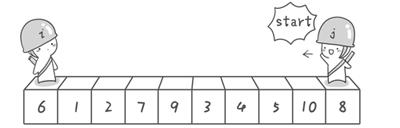2.哨兵j从右开始找比基位6小的数“5”，哨兵i比基位6大的数“7”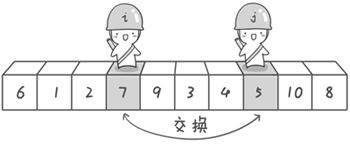3.交换“5”跟“7”的位置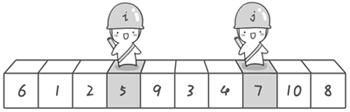4.继续重复1-3的步骤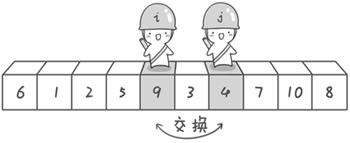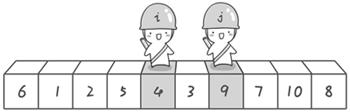5.哨兵j继续往左走，找到了比基位6小的数“3”后停止。然后哨兵i往右走找比基位6大的数。正好哨兵i和哨兵j相遇，所有的数都已经和6比较完了。这时候哨兵i的位置和基位“6”交换。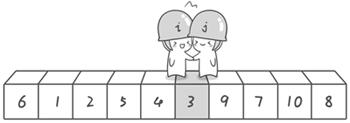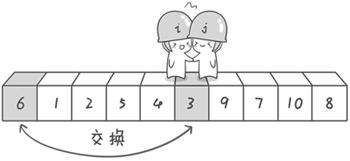6.交换完后“6”左边的都比“6”小，“6”右边的都比“6”大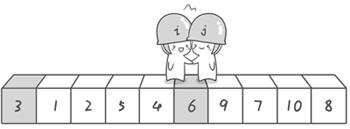7.继续按上面的步骤比较“6”左边的3，1，2，5，4和右边的9，7，10，8直到所有的数都为有序状态
快速排序的Java代码

``````public class Quick {
private static void sort(int[] intArrays ,int left,int right) {
//如果左索引大于右索引，直接返回
if(left > right){
return;
}
int i = left ;
int j = right;
int temp = intArrays[left];//设置基准值，将最左端元素作为基准值
while(i != j){
//往左移位，直到小于temp
while(i<j && intArrays[j]>=temp){
j--;
}
//往右移位，直到大于temp
while(i<j && intArrays[i]<=temp){
i++;
}
if(i < j){
//如果i<j,也就是说i和j还没相遇时，交换彼此的数据
NumberUtils.exchange(intArrays,i,j);
}
}
//当哨兵i与哨兵j相遇时退出循环，将哨兵i与基位交换位置
NumberUtils.exchange(intArrays,left,i);
//下一次迭代
sort(intArrays,left,i-1);//左半边
sort(intArrays,j+1,right);//右半边
}

/** * 执行入口，intArrays：待排序的数组，displaySort：是否显示排序前和排序后的内容。 * @author linyuanhuang * @Date 11:50 2017/12/28 * @return void */
public static void run(int intArrays[],boolean displaySort){
//克隆一份数组
int arrays[]= intArrays.clone();
// 判断是否需要显示排序前的内容
if(displaySort){
NumberUtils.display(arrays,1);
}
// 记录开始时间
long startTime=System.currentTimeMillis();
sort(arrays,0,arrays.length-1);
// 记录结束时间
long endTime=System.currentTimeMillis();
// 判断是否需要显示排序前的内容
if(displaySort){
NumberUtils.display(arrays,2);
}
System.out.println("快速排序用时："+(endTime-startTime)+"毫秒");
}
/** * 测试排序用的主方法 */
public static void main(String[] args){
//数组长度
int length=30000;
//最大值
int max =100000000;
//是否打印排序后的内容
boolean display=true;
//随机获取的排序数组
int intArrays[]= NumberUtils.getRandomArs(length,max);
//快速排序
Quick.run(intArrays,display);
}
}``````

运行main方法，终端输出

``````排序前：43139629 7649925 81580157 40853496 33416709 66768707 92323968 94560370 19094255 ......84317262 13986402 3498445 28911241 28768513 583418 73503650 17522821 70353692 97132323

归并排序

归（递归）并（合并）排序采用了分治策略（divide-and-conquer），就是将原问题分解为一些规模较小的相似子问题，然后递归解决这些子问题，最后合并其结果作为原问题的解。
归并排序将待排序数组A[1..n]分成两个各含n/2个元素的子序列，然后对这个两个子序列进行递归排序，最后将这两个已排序的子序列进行合并，即得到最终排好序的序列。具体排序过程如下图所示：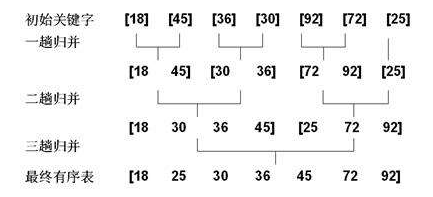归并排序的Java代码

``````public class Merge {
/** * 临时数组空间 */
private static int[] tmpArray;
/** * 排序--分解为一些规模较小 * @author linyuanhuang * @Date 12:01 2017/12/28 * @return void */
private static void sort(int[] a, int left, int mid, int right) {
int i = left; //左数组下一个要进行比较的元素的索引
int j = mid + 1; //右数组下一个要进行比较的元素的索引
int N = right + 1; //本次归并的元素数目

for (int k = left; k <= right; k++) {
if (i > mid) {  //左数组元素已全比较完
tmpArray[k] = a[j++];
} else if (j > right) { //右数组元素已全比较完
tmpArray[k] = a[i++];
} else if (a[j] < a[i]) { //右数组元素小于左数组
tmpArray[k] = a[j++];
} else {  //右数组元素大于等于左数组
tmpArray[k] = a[i++];
}
}
//归并完成后，再复制回原数组
for (int k = left; k < N; k++) {
a[k] = tmpArray[k];
}
}
/** * 归并排序开始入口 * @author linyuanhuang * @Date 11:57 2017/12/28 * @return void */
public static void sort(int[] a) {
int N = a.length;
tmpArray = new int[N+1]; //用于暂时存放比较后的元素
merge(a, 0, N - 1);
}
/** * 递归方法 * @author linyuanhuang * @Date 12:04 2017/12/28 * @return void */
private static void merge(int[] a, int left, int right) {
//左索引大于等于右索引直接返回
if (left >= right) {
return;
}
//一分为二
int mid = (left + right) / 2;
//递归一分为二左边的队列
merge(a, left, mid);
//递归一分为二右边的队列
merge(a, mid+1, right);
//排序
sort(a, left, mid, right);
}

/** * 执行入口，intArrays：待排序的数组，displaySort：是否显示排序前和排序后的内容。 * @Author linyuanhuang * @Date 2017/12/22 15:11 * @return void */
public static void run(int intArrays[],boolean displaySort){
//克隆一份数组
int arrays[]= intArrays.clone();
// 判断是否需要显示排序前的内容
if(displaySort){
NumberUtils.display(arrays,1);
}
// 记录开始时间
long startTime=System.currentTimeMillis();
sort(arrays);
// 记录结束时间
long endTime=System.currentTimeMillis();
// 判断是否需要显示排序前的内容
if(displaySort){
NumberUtils.display(arrays,2);
}
System.out.println("归并排序用时："+(endTime-startTime)+"毫秒");
}
}
/** * 测试排序用的主方法 */
public static void main(String[] args){
//数组长度
int length=30000;
//最大值
int max =100000000;
//是否打印排序后的内容
boolean display=true;
//随机获取的排序数组
int intArrays[]= NumberUtils.getRandomArs(length,max);
//归并
Merge.run(intArrays,display);
}
}``````

运行main方法，终端输出

``````排序前：57743991 26662091 86541142 1246814 1386809 51656969 28286793 30436571 97487893 ......20682423 59991342 19182409 51239835 5409807 47490219 76499337 42071620 8118816 30246698

基数排序

1、关键字可分解；
2、记录的关键字位数较少，如果密集更好；
3、如果是数字时，最好是无符号的，否则将增加相应的映射复杂度，可先将其正负分开排序。
初始化：构造一个10*n的二维数组，一个长度为n的数组用于存储每次位排序时每个桶子里有多少个元素。
循环操作：从低位开始（我们采用LSD的方式），将所有元素对应该位的数字存到相应的桶子里去（对应二维数组的那一列）。然后将所有桶子里的元素按照桶子标号从小到大取出，对于同一个桶子里的元素，先放进去的先取出，后放进去的后取出（保证排序稳定性）。这样原数组就按该位排序完毕了，继续下一位操作，直到最高位排序完成。
待排序的数组：70, 34, 65, 24, 48, 32, 88, 16, 38, 81
按个位排序：

70 81 32 34 65 16 48
24 88
38

按个位排完之后的顺序：70，81，32，34，24，65，16，48，88，38
按十位排序：

16 24 32 48 65 70 81
34 88
38

按十位排完之后的顺序：16，25，32，34，38，48，65，70，81，88
堆排序的java代码：

``````public class Radix {
public static void sort(int[] intArrays,int max) {
int n=1;//代表位数对应的数：个位、十位、百位、千位..直到小于max的最大位数
int k=0;//保存每一位排序后的结果用于下一位的排序输入
int length=intArrays.length;
int[][] bucket=new int[length];//排序桶用于保存每次排序后的结果，这一位上排序结果相同的数字放在同一个桶里
int[] order=new int[length];//用于保存每个桶里有多少个数字
while(n<max)
{
for(int num:intArrays) //将数组array里的每个数字放在相应的桶里
{
int digit=(num/n)%10;
bucket[digit][order[digit]]=num;
order[digit]++;
}
for(int i=0;i<length;i++)//将前一个循环生成的桶里的数据覆盖到原数组中用于保存这一位的排序结果
{
if(order[i]!=0)//这个桶里有数据，从上到下遍历这个桶并将数据保存到原数组中
{
for(int j=0;j<order[i];j++)
{
intArrays[k]=bucket[i][j];
k++;
}
}
order[i]=0;//将桶里计数器置0，用于下一次位排序
}
n*=10;//扩大位数，如从个位扩大到十位
k=0;//将k置0，用于下一轮保存位排序结果
}
}
/** * 执行入口，intArrays：待排序的数组，displaySort：是否显示排序前和排序后的内容。 * @Author linyuanhuang */
public static void run(int intArrays[],int max,boolean displaySort){
//克隆一份数组
int arrays[]= intArrays.clone();
// 判断是否需要显示排序前的内容
if(displaySort){
NumberUtils.display(arrays,1);
}
// 记录开始时间
long startTime=System.currentTimeMillis();
sort(arrays,max);
// 记录结束时间
long endTime=System.currentTimeMillis();
// 判断是否需要显示排序前的内容
if(displaySort){
NumberUtils.display(arrays,2);
}
System.out.println("基数排序用时："+(endTime-startTime)+"毫秒");
}
/** * 测试排序用的主方法 */
public static void main(String[] args){
//数组长度
int length=30000;
//最大值
int max =100000000;
//是否打印排序后的内容
boolean display=true;
//随机获取的排序数组
int intArrays[]= NumberUtils.getRandomArs(length,max);
//基数排序
}
}``````

运行main()方法：

``````排序前：57333024 46035713 59041372 78828841 11511663 53444973 7926947 73163026 85022558 ......18902011 21833472 33961470 32584321 19164487 74110617 26777609 90189102 74077757 81084518

性能比较

乱序

先执行三次下面的main()方法，看看平均值是否在正常范围：

``````public static void main(String[] args){
//数组长度
int length=30000;
//最大值
int max =100000000;
//是否打印排序后的内容
boolean display=false;
//随机获取的排序数组
int intArrays[]= NumberUtils.getRandomArs(length,max);
//冒泡排序
Bubble.run(intArrays,display);
//选择排序
Selection.run(intArrays,display);
//插入排序
Insertion.run(intArrays,display);
//希尔排序
Shell.run(intArrays,display);
//归并
Merge.run(intArrays,display);
//快速
Quick.run(intArrays,display);
//堆排序
Heap.run(intArrays,display);
//基数排序
}``````

第一次运行结果

``````冒泡排序用时：2368毫秒

第二次运行结果

``````冒泡排序用时：2437毫秒

第三次运行结果

``````冒泡排序用时：2603毫秒

升序

``````排序前：4771 6772 8312 9057 10991 12544 16315 16764 29695 ......99967869 99972597 99974922 99976218 99976267 99980394 99985293 99987745 99993483 99997019

降序

``````排序前：99997596 99996734 99990734 99989287 99982918 99977264 99975157 99973732 99961619 ......33090 29778 25590 18581 11443 10509 5215 4429 3013 2184

如果对排序的顺序不确定的情况下，建议现将待排序的元素随机后再进行排序。或者选择归并、堆排序这类时间复杂度比较稳定的排序

时间复杂度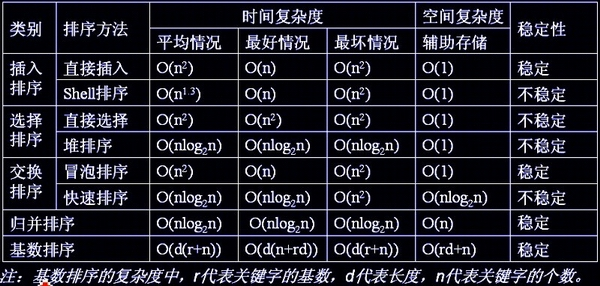复杂度函数时间大小比较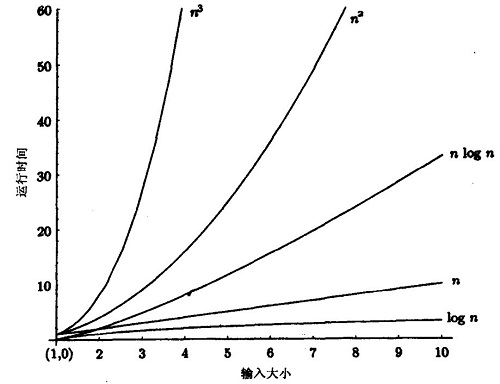GitHub地址

https://github.com/lyhkmm/algorithm

• 浏览(261)
• 浏览(215)
• 浏览(187)
• 浏览(177)
• 浏览(170)
• 浏览(133)
• 浏览(133)
• 浏览(128)
• 浏览(125)
• 浏览(123)